# Chain Rule Derivative in Machine Learning : Explained

Machine Learning has evolved to become one of the most talked-about and researched fields in the current years, and for all the good reasons. New models and applications of machine learning are being discovered every day, and researchers around the globe are working towards the next big thing.

As a result, there has been an increased interest in professionals from varied backgrounds to switch to machine learning and be a part of this ongoing revolution. If you’re one such machine learning enthusiast looking to take their first steps, let’s tell you that it begins with understanding the basics of mathematics and statistics before anything else.

One such vital topic in Mathematics that is highly relevant to machine learning is derivatives. From your basic understanding of calculus, you’d remember that the derivative of any function is the instantaneous rate of change of that function. In this blog, we’ll dive deeper into derivatives and explore the chain rule. We’ll see how a particular function’s output changes when we change some independent variables in the equation. With the knowledge of chain rule derivatives, you’ll be able to work on differentiating more complex functions that you are sure to encounter in machine learning.

## Understanding the Chain Rule Derivative

The chain rule is essentially a mathematical formula that helps you calculate the derivative of a composite function. A composite function is one that is composed of two or more functions. So, if f and g  are two functions, then the chain rule would help us find the derivative of composite functions such as f o g or g o f.

Considering the composite function f o g, here’s what the chain rule derivative would look like: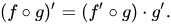The above rule can also be written as: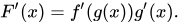Where the function F is the composition of f and g, in the form of f(g(x)).

Now, suppose we have three variables such that the third variable (z) depends on the second variable (y), which in turn depends on the first variable (x). In that case, the chain rule derivative would look something like this: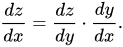In terms of deep learning, this is also the formula regularly used to solve backpropagation problems. Now, since we mentioned that z depends on y and y on x, we can write z = f(y) and y = g(x). This substitution would modify our differential equation in the following manner: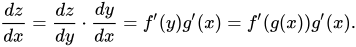Now, let’s look at some examples of chain rule derivatives to better understand the maths behind them.

## Examples and Applications of Chain Rule Derivative

Let us take a well-known example from Wikipedia to understand the chain rule derivative in a better manner. Assume you’re taking a free fall from the sky. The atmospheric pressure that you encounter during the fall will constantly keep changing. Here is a graph that plots this change of atmospheric pressure with elevation levels: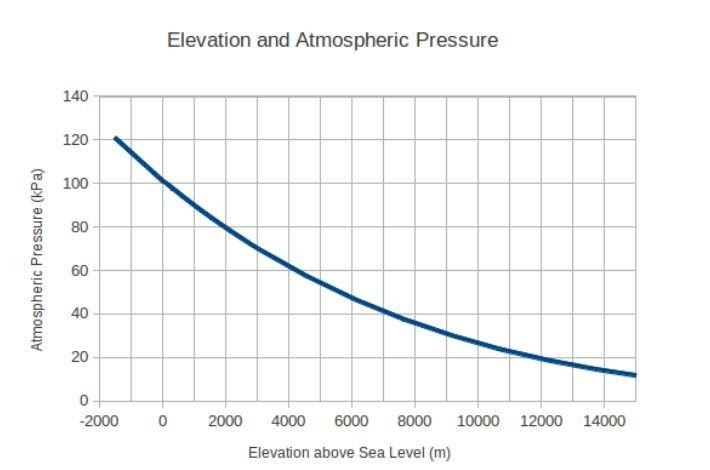Suppose your fall started at 4000 meters above sea level. Initially, your velocity was zero, and the acceleration value was 9.8 meters per second squared due to gravity.

Now, let’s compare this situation with the previous chain rule method. In this example, we’ll be using the variable ‘t’ for time instead of x.

Then, the variable y = g(t), which tells the distance travelled since the beginning of the fall, can be given as:

g(t) = 0.5*9.8t^2

And, the height from the sea level can be given by a variable ‘h’, which will be equal to 400-g(t).

Assume that, based on a model, we can also write the function of the atmospheric pressure at any height h as:

f(h) = 101325 e−0.0001h

Now, you can distinguish between the two equations based on their dependant variables to get the following results:

g′(t) = −9.8t,

Here, g’(t) tells the value of your velocity at any time t.

f′(h) = −10.1325e−0.0001h

Here, f′(h) is the rate of change in atmospheric pressure with respect to height h. Now, the question is can we combine these two equations and derive the rate of change of atm pressure wrt the time? Let’s see using the chain rule: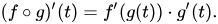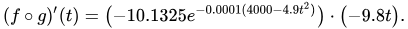The final equation that we’ve got provides us with the changing rate of the atmospheric pressure in relation to the time passed since fall. In terms of machine learning, neural networks constantly need weight updates concerning the neuron’s error in prediction. The chain rule helps adjust these weights and take the machine learning model closer to the correct output.

## Conclusion

As you can see, the chain rule is beneficial for many purposes. Especially when it comes to machine learning or deep learning, the chain rule finds a lot of use in updating the weights of the neurons and improving the overall efficiency of the model.

Now that you’re aware of the basics of the chain rule go ahead and try a few problems on your own. Lookup a few composite functions and try to find their derivatives. The more you practice, the clearer your concepts will get, and the easier it’ll be for you to train your machine learning models! That said, if you’re a machine learning enthusiast but struggling to take your first steps in this field, upGrad has your back!

Our Executive PG Programme in Machine Learining & AI is offered in collaboration with IIIT-Bangalore and gives you the choice of six industry-relevant specialisations. The course starts from the ground level and takes you to the apex while providing you with 1-on-1 support from industry experts, a strong peer group of students, and 360-degree career support.

## Lead the AI Driven Technological Revolution

EXECUTIVE PG PROGRAM IN MACHINE LEARNING AND ARTIFICIAL INTELLIGENCE
Apply Now

## 0 replies on “Chain Rule Derivative in Machine Learning : Explained”

### Our Trending Machine Learning Courses